# Calculating Differential Proportionality

## Introduction

In this vignette, we use a real dataset to show how we can apply differential proportionality analysis to understand RNA-seq count data. We place a particular emphasis here on documenting the several differential proportionality measures available through this package. This vignette discusses the propd method.

## Theory

Let us consider two non-zero positive integer vectors, $$X$$ and $$Y$$, measuring the relative abundance of raw sequence counts. One way to understand whether two vectors associate with one another is to consider the variance of the log-ratios (VLR), a compositionally valid measure of association that makes up part of the definition of the proportionality metrics $$\phi$$ and $$\rho$$. Although we can calculate VLR using log-ratio transformed data, we see that in this case the denominator factor (e.g., the geometric mean or unchanged reference) cancels. As such, we can use these counts without any transformation:

$\textrm{VLR(X, Y)} = \textrm{var}(\log(\textrm{X} - \textrm{Y}))$

The propd method uses the VLR to test for differential proportionality. Recall that the reason we do not use the VLR for proportionality analysis is that we cannot compare the VLR for one pair against the VLR for another pair (thus why we defined $$\phi$$ and $$\rho$$ as a modification to the VLR that gives it scale). However, in differential proportionality analysis, we extract the fraction of variance (interpretted as the residual variance of proportional features) where proportionality holds in only one (or both) of two groups.

We consider here two forms of differential proportionality. The first, which we call disjointed proportionality, considers the case where the proportionality of a pair holds in both groups, but the ratio between the partners changes between the groups (i.e., the slope of the proportionality changes). The second, which we call emergent proportionality, considers the case where there is proportionality in only one of the groups (i.e., the strength of the proportionality changes).

Given two groups, sized $$k$$ and $$n - k$$, we define $$\theta_d$$, a measure of disjointed proportionality, as the pooled (weighted) VLR within the two groups divided by the total VLR:

$\theta_d(\textrm{X}, \textrm{Y}) = \frac{(k-1)\textrm{VLR}_1 + (n-k-1)\textrm{VLR}_2}{(n-1)\textrm{VLR}}$

Likewise, we define $$\theta_e$$, a measure of emergent proportionality, as the fraction of variance that remains when subtracting the fraction of the dominating group variance:

$\theta_e(\textrm{X}, \textrm{Y}) = 1 - \frac{\mathrm{max}[(k-1)\textrm{VLR}_1,(n-k-1)\textrm{VLR}_2]}{(n-1)\textrm{VLR}}$

## Handling of zero values

Note that as a consequence of using the logarithm, we cannot compute VLR in the setting of zero counts. How to best handle zeros in compositional data analysis remains an area of active research. By default, propr and propd take the simplest approach of replacing all zeros with the value one. Analysts should carefully evaluate the effect that this has on their results.

In addition, the propd method offers the option to use a transformation to approximate VLR based on a parameter $$\alpha$$ that is usually close to zero. We define aVLR as an approximation of VLR, used in place of VLR in any $$\theta$$ equation:

$\textrm{aVLR(X, Y)} = \frac{\sum\left(\frac{\textrm{X}^{\alpha}}{\textrm{mean}(\textrm{X}^{\alpha})} - \frac{\textrm{Y}^{\alpha}}{\textrm{mean}(\textrm{Y}^{\alpha})}\right)^2}{(n-1)\alpha^2}$

## False discovery rate

The propd method, due to the unwieldy number of pairs, does not return a vector of p-values like most statistical tests. Instead, it calculates the false discovery rate (FDR) directly using permutations of the group assignments to generate an empiric distribution of $$\theta$$ values specific to the supplied data. With this, for each arbitrary cutoff of $$\theta$$, we can calculate a FDR based on the average random number of pairs with $$\theta<\textrm{cutoff}$$ divided by the observed number of pairs with $$\theta<\textrm{cutoff}$$.

Alternatively, it is possible to calculate the FDR based on the F-statistic, a procedure which we will discuss in more detail in a future vignette:

$F = (n-2)\frac{1-\theta_d}{\theta_d}$

## Implementation

The propd function estimates differential proportionality by calculating $$\theta$$ for all feature pairs, with or without the aid of the transformed ($$\alpha$$) aVLR. This function takes the following arguments as input and returns a propd object as a result:

• counts: a matrix of $$n$$ samples (as rows) and $$d$$ features (as columns)
• group: an $$n$$-dimensional vector corresponding to subject labels
• alpha: an optional argument to trigger and guide transformation
• p: the total number of permutations used to estimate FDR
• cutoff: an optional vector of cutoffs for which to estimate FDR

Below, we run propd using the iris data set.

library(propr)
data(iris)
keep <- iris\$Species %in% c("setosa", "versicolor")
counts <- iris[keep, 1:4] * 10
group <- ifelse(iris[keep, "Species"] == "setosa", "A", "B")
pd <- propd(counts, group, alpha = NA, p = 100, cutoff = NA)

The resultant propd object contains both $$\theta_d$$ and $$\theta_e$$ metrics, although only $$\theta_d$$ is active by default. While a $$\theta$$ is active, it forms the basis for permutation testing (i.e., FDR estimation) and visualization. Users can easily change which $$\theta$$ is active using the functions setDisjointed and setEmergent.

theta_d <- setDisjointed(pd)
theta_e <- setEmergent(pd)

Once the $$\theta$$ of interest is active, the user can calculate FDR using the updateCutoffs function. By default, providing a cutoff argument directly to the propd function will run updateCutoffs for $$\theta_d$$.

theta_d <- updateCutoffs(theta_d, cutoff = seq(0.05, 0.95, 0.3))
## |------------(25%)----------(50%)----------(75%)----------|
theta_e <- updateCutoffs(theta_e, cutoff = seq(0.05, 0.95, 0.3))
## |------------(25%)----------(50%)----------(75%)----------|

In order to reduce RAM overhead, the propd object never stores the intermediate $$\theta$$ values for permutation testing. However, when a propd object is created, it contains all the randomized group assignments needed for permutation testing, meaning that each updateCutoffs run effectively uses the same random seed. One could exploit the data contained in pd@permutes to reproduce the intermediate calculations if needed.

## Sample data

To understand differential proportionality, we use two propd objects, called pd.d (for $$\theta_d$$) and pd.e (for $$\theta_e$$), built from the bundled caneToad.counts RNA-Seq data (Rollins 2015). Specifically, we use the results of the propd function as applied to cane toad transcripts with at least 40 counts in all 20 samples (after removing any transcripts with 0 counts), subsetted to include only pairs with the top 1000 smallest $$\theta$$.

Note that in this vignette, we never apply updateCutoffs to either of these data objects. When estimating FDR, it is necessary to use an unfiltered propd object to keep estimations unbiased.

data(pd.d, package = "propr") # top 1000 disjointed pairs
data(pd.e, package = "propr") # top 1000 emergent pairs

## Disjointed proportionality

We begin now by looking at disjointed proportionality in more detail. Based on its definition, we see that low values of $$\theta_d$$ select pairs where the total VLR far exceeds the weighted sum of the within-group VLRs. Often, the within-group VLRs are about the same size. However, this is not a requirement so long as the within-group VLRs are both small compared to the total VLR.

Below, we use the shale function, analogous to the slate function, to tabulate important pair-wise measurements. Then, we show a scatter plot of the abundance for features “39” and “37”, as colored by the experimental group, with the slopes of the trend lines equal to the ratio means.

tab <- shale(pd.d)
head(round(tab[, c("Partner", "Pair", "theta", "LRV", "LRV1", "LRV2", "LRM1", "LRM2")], 2))
##     Partner Pair theta  LRV LRV1 LRV2  LRM1  LRM2
## 76       13   10  0.19 0.24 0.03 0.07  1.77  0.90
## 191      21    1  0.17 0.14 0.03 0.02 -1.66 -2.33
## 385      29    7  0.20 0.26 0.05 0.06 -4.21 -5.11
## 740      39   37  0.12 0.43 0.05 0.06 -0.42  0.78
## 778      40   37  0.19 0.51 0.15 0.05 -0.62  0.64
## 899      43   38  0.18 0.49 0.12 0.08  0.89 -0.34
plot(pd.d@counts[, 39], pd.d@counts[, 37], col = ifelse(pd.d@group == "WA", "red", "blue"))
grp1 <- pd.d@group == "WA"
grp2 <- pd.d@group != "WA"
abline(a = 0, b = pd.d@counts[grp1, 37] / pd.d@counts[grp1, 39], col = "red")
abline(a = 0, b = pd.d@counts[grp2, 37] / pd.d@counts[grp2, 39], col = "blue")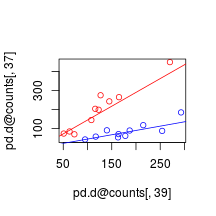Here, we see that these two features change proportionally across the samples within each group (as expected based on their small values of $$\textrm{VLR}_1$$ and $$\textrm{VLR}_2$$). However, when ignoring the group labels, the relationship between these two features appears noisy. It seems as if the experimental condition has moved the set point around which the transcripts behave proportionally (i.e., the y-intercept has moved). In other words, we see that, while “37” (y-axis) has increased in expression relative to “39” (x-axis), “37” is no less coordinated with “39”. This change in ratio abundance is apparent when viewed through another projection.

plot(pd.d@counts[, 37] / pd.d@counts[, 39],
col = ifelse(pd.d@group == "WA", "red", "blue"))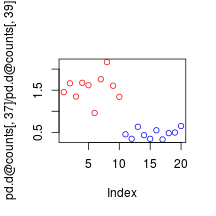This figure shows a clear difference in the ratio abundances between the groups. It also highlights the analogy between disjointed proportionality and differential expression, although the interpretation of differentially abundant ratios differs considerably. Possible biological explanations for this event might include a reduction in the amount of mRNA degradation, a change in isoform splice bias, or an increase in the binding affinity (i.e., $$K_a$$) of a transcriptionally relevant co-factor.

## Emergent proportionality

In contrast, emergent proportionality has more in common with a test for differences in correlation coefficients. That is, emergent proportionality occurs when a pair is proportional in one group but not the other, such that the group with no proportionality contributes most of the total variance.

Below, we use the shale function again to tabulate important pair-wise measurements. Then, we show a scatter plot of the abundance for features “106” and “2”, as colored by the experimental group, with the slopes of the trend lines equal to the ratio means.

tab <- shale(pd.e)
head(round(tab[, c("Partner", "Pair", "theta", "LRV", "LRV1", "LRV2", "LRM1", "LRM2")], 2))
##      Partner Pair theta  LRV LRV1 LRV2  LRM1  LRM2
## 666       37   36  0.04 0.22 0.44 0.02 -0.34 -0.39
## 1364      53   38  0.04 0.29 0.59 0.02 -0.87 -0.91
## 2137      66   57  0.05 0.22 0.02 0.44  1.10  1.06
## 3269      82   29  0.05 0.14 0.28 0.01 -1.33 -1.36
## 5462     106    2  0.05 0.43 0.87 0.04 -0.33 -0.36
## 7783     126   33  0.04 0.13 0.27 0.01 -0.19 -0.19
plot(pd.e@counts[, 106], pd.e@counts[, 2], col = ifelse(pd.d@group == "WA", "red", "blue"))
grp1 <- pd.e@group == "WA"
grp2 <- pd.e@group != "WA"
abline(a = 0, b = pd.e@counts[grp1, 2] / pd.e@counts[grp1, 106], col = "red")
abline(a = 0, b = pd.e@counts[grp2, 2] / pd.e@counts[grp2, 106], col = "blue")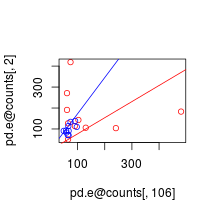Here, we see that these two features change proportionally across the samples within one group but not the other. Moreover, when ignoring the group labels, we see that one group happens to dominate the total VLR. In other words, the experimental condition appears to have removed the coordination between the transcripts. However, this has happened here without much change in the average abundance ratio.

plot(pd.e@counts[, 2] / pd.e@counts[, 106],
col = ifelse(pd.d@group == "WA", "red", "blue"))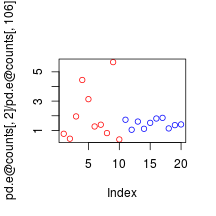This figure confirms that the feature pair varies far more in one group that the other, all while the mean ratio abundances do not change considerably. Note that an increase in $$\theta_e$$ tends to impart a decrease in $$\theta_d$$. Precisely, $$\theta_e$$ relates to $$\theta_d$$ via the function:

$\vartheta_\mathrm{e}=1-\vartheta + \frac{\mathrm{min}[(k-1)\textrm{VLR}_1,(n-k-1)\textrm{VLR}_2]}{(n-1)\textrm{VLR}}$

From this, we establish the inequality:

$1-\vartheta\le\vartheta_\mathrm{e}\le1-\vartheta/2,$

Interestingly, one can use $$1 - \theta_e$$ for a stricter definition of disjointed proportionality. This is implemented in propd as $$\theta_f$$, which one can set active using the setActive function.

pd.f <- setActive(pd, what = "theta_f")
## Use 'updateCutoffs' to refresh FDR.

## Disproportionality networks

A good first step in understanding $$\theta$$ begins with an examination of the disproportionality network. The plot method provides an easy way to build a network such that each circular “node” indicates a transcript while each connecting “edge” indicates an indexed pair (i.e., $$\theta < cutoff$$). Providing a value of $$[0, 1]$$ to the cutoff argument will index pairs based on a maximum value of $$\theta$$. Providing an integer greater than $$1$$ to the cutoff argument will index the top $$N$$ pairs as ranked by $$\theta$$. Note that setting d3 = TRUE will have the rgl package render the network in 3D.

g <- plot(pd.d, cutoff = 1000)
## Red: Pair has higher LRM in group WA than in group QLD
## Blue: Pair has lower LRM in group WA than in group QLD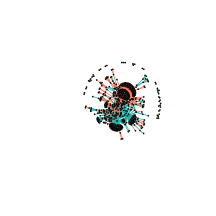For disjointed proportionality networks, red edges show an increase in log-ratio means in Group 1 compared to Group 2 (i.e., increased ratio abundance in Western Australia toads) while blue edges show a decrease in log-ratio means in Group 1 compared to Group 2 (i.e., decreased ratio abundance in Western Australia toads). Importantly, we see that a small number of transcripts participate in a large number of differentially proportional pairs.

g <- plot(pd.e, cutoff = 1000)
## Red: Pair has nearly absent LRV in group WA than in group QLD
## Blue: Pair has nearly total LRV in group WA than in group QLD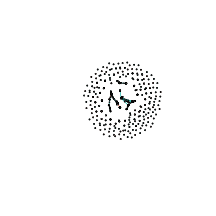For emergent proportionality networks, red edges show an emergence of proportionality in Group 1 compared to Group 2 (i.e., sudden coordination in Western Australia toads) while blue edges show a lack of proportionality in Group 1 compared to Group 2 (i.e., no coordination in Western Australia toads). The architecture of this network is more sparse than the other, making it hard to interpret. However, when viewed in 3D, we can see a few clusters of transcripts that all gained or lost coordination together.

Note that with the appropriate cutoff, a network will project all $$\theta$$ pairs for a given FDR. However, we have routinely found that too many pairs remain when using cutoffs with an acceptable FDR. In this case, we recommend stepping through a number of arbitrarily low cutoffs to find one that produces a representative topology that is computationally tractable and human-interpretable.

## Visualizing log-ratio abundance

The slice function shows the sample-wise distribution of log-ratio abundances for each pair relative to a reference feature. The reference argument can specify any feature by name, although users would likely choose a “hub” as the reference. Note that it is always possible for a “hub” to have changed relative to its neighbors or for a “hub” and its neighbors to have changed simultaneously.

slice(pd.d, reference = "c19327_g2_i3")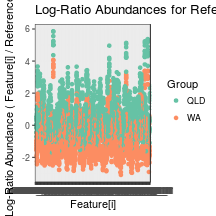slice(pd.e, reference = "c27054_g5_i1")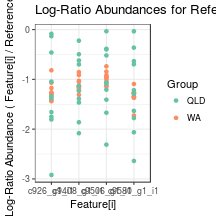## Integrating propd with propr

This package also allows users to integrate the results of proportionality analysis with those from differential proportionality analysis through a union of a propr network with a propd network. We do this with the plot method by passing an indexed propr object to the propr argument. We recommend the companion vignette, “How Proportional Is Proportional Enough?” for selecting an appropriate cutoff of $$\rho$$. Note that, because CRAN imposes limits on the size of bundled data, as well as the run-time of vignette assembly, we cannot render this figure here. Instead, we provide the code only.

data(caneToad.counts)
keep <- apply(caneToad.counts, 2, function(x) sum(x >= 40) >= 20 & all(x != 0))
plot(pd.e, propr = rho, d3 = TRUE)